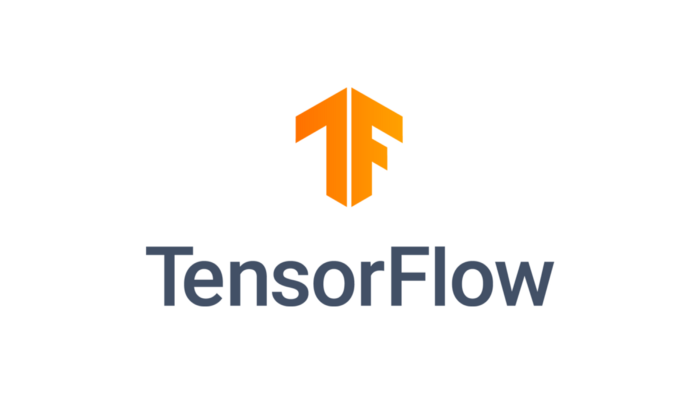# Basics of TensorFlow 1.x part 2

Original article can be found here (source): Artificial Intelligence on Medium`%tensorflow_version 1.ximport tensorflow as tfa = tf.constant(21, tf.int16, name = 'x')b = tf.constant(0.098, tf.float32, name = 'y')c = tf.constant(78.90, name = 'z')print(a, b, c)operation1 = tf.add(b, c)print(operation1)operation2 = tf.sqrt(b)sess = tf.Session()print(sess.run(operation1))print(sess.run(operation2))`

Shorthand operations can also be used in place of TensorFlow operations. For example, operation3 = a * b is the shorthand operation for the method tf.multiply(a, b)